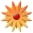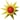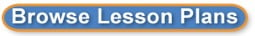Manipulating Graphs

Page Views: 3019

Email This Lesson Plan to Me
Overall Rating:(5.0 stars, 1 ratings)

 Keywords: graphing, slopes, intercepts Subject(s): Grades 7 through 8 School: Olive Vista Middle School, Sylmar, CA Planned By: Brent Obillo Original Author: Brent Obillo, Sylmar
-Students are given a series of real life situations
-Angel gets \$2 a day and he started with \$3
-Fatima gets \$6 a day and started with \$1
-Tina spends \$3 a day and started with \$21

-Have students create the equations for each situation
-Have students graph the "mother function" y=x
-Have students graph (on the same axis) the equations for each of the different situations
-"How does the amount they get each day affect the graph?"
-"How does their starting amount affect the graph?"
-"Tina spent money, how do we see this in the graph?"
-Have students generalize how the slope changes the graph and the y-intercept affects the graph.
 Comments If you being the modeling of graph analysis with this, it can be extended to how a,b, and c affect a quadratic graph as well as how amplitude and period can be chanegd in periodic functions. Materials: Graphing